Math Exams

# 40 Interesting Facts About Maths

48.2k views

 1 Introduction 2 Math Facts! 40 Interesting Facts About Maths 3 Conclusion 5 Frequently Asked Questions (FAQs) 6 External References

### 20 November 2020

Did you know?

Mathematics is a very interesting subject. From its rich history to different tricks and tips, mathematics lays down a number of amazing facts to help students get involved with this subject in a better way.You may also like:

## Math Facts! 40 Interesting Facts About Maths

In this article, we are listing down 40 amazing facts about maths. These maths fun facts will help students get involved with this subject in a better way.

1. There are types of interesting numbers in mathematics. One such number is hundred. The number 100 does not actually mean 100. It is derived from the Old Norse word "hundredth," which actually means 120.1. The number 2 is the smallest prime number. It is also the only even prime number. The digit 1 is considered to be prime to every other number. Therefore, we take 2 as the smallest.

2. We all have heard about the Pythagoras theorem. But there is also something which is known as Pythagoras constant. The square root of 2 i.e. 1.41 was the first rational number to be discovered which is known as the Pythagoras constant.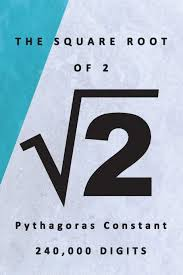1. Romans didn't consider 0 to be a number at all. Therefore, there are in total zero zeros in Roman numerals.

2. The idea of zero was invented by an Indian astronomer and mathematician Brahmagupta in around 600 A.D. He contributed a lot to mathematics and astronomy and is known for explaining how to find the cube and cube-root of an integer.

3. Roman numerals only have seven different letters which form the entire number system:  I, V, X, L, C, D, and M.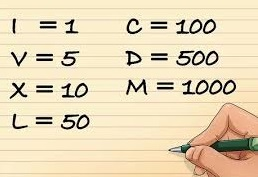1. Mathematically, an even number is a number that can be divided into two smaller numbers which are actually whole numbers.

2. There is only one number in the entire Hindu Arabic number system which can be spelled with the same number of letters as itself. That number is four.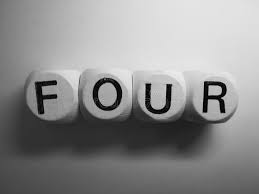1. If you take any number multiplied by three and sum the digits in the answer, then the answer you receive after adding the digits will always be divisible by three. For example: 3 x 4 = 12 or, 1 + 2 = 3 or, 3 / 3 = 1

2. A perfect number refers to a positive integer that is equal to the sum of its positive divisors. By this rule, 6 is the smallest perfect number. The next perfect number is 28.

3. We follow the decimal system number which has 10 digits from 0 to 9. It is also known as the Hindu Arabic numeral system. It was discovered more than 1000 years ago.

4. Pi is also known as the ratio of circumference to the diameter of a circle. It is a special number that is irrational. There is a designated day, called Pi day, that we celebrate on March 14.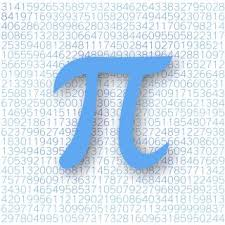1. Leonardo Fibonacci from Pisa, in the 13th century, discovered the Fibonacci sequence. Starting at 0 and 1, this sequence is created as the sum of the two preceding numbers, For example, 0, 1, 1, 2, 3, 5, 8, 13, 21, 34, …

2. 9 is also known as the magic number. This is because if you multiply a number by 9 and add all the digits of the new number together, the sum will always add up to 9. For example: 8 x 9 = 72 or, 7 + 2 = 9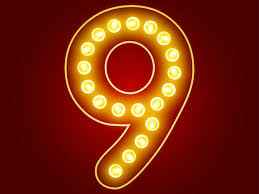1. Very often, the number 1 is confused as a prime number but by dividing 1 by 1, you get 1. Nothing has been divided.

2. The power of exponential growth is shocking. You can actually reach the moon by folding a paper of 0.01mm 45 times.

3. If you walked up on the street and asked someone about their favorite number, there is almost a 10% chance that they will say number seven.

4. 13 is regarded as an unlucky number. But in Alex Bellos' research, it turned out to be the most popular two-digit number.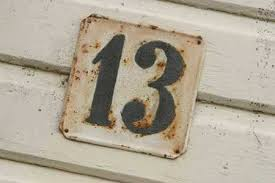1. Googol means 1 followed by 100 zeroes. A googolplex is 1 followed by a googol zeros. It is hard to imagine how long this number would be.

2. Denver is known as the mile-high city because it has an elevation of precisely 5280 feet – the length of a mile.

3. Number four is viewed with superstition and distrust and much of East Asia. This is known as tetra phobia. This is because the word four sounds similar to that in a number of Asian languages.1. Teenagers texting in Thailand will send the digits 555 to indicate that something is funny. In the Thai language, 5 is pronounced as ha which when translated becomes hahaha.

2. The majority of the months have 31 days in a calendar. Only four months have 30 days i.e. April, June, September, and November. February typically only has 28 days, with the exception of leap years.

3. In a sample of 23 people, there are 50% chances that two of them will share the same birthday. This is known as the birthday problem.

4. Seven is the most significant number across religions and cultures. For example, seven colors in a rainbow, seven days in a week, seven notes on the musical skill, etc.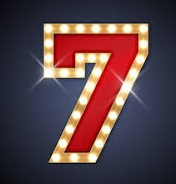1. The history of “inch”. In Britain, the unit of length was the barleycorn. After 1066, 1 inch was equal to 3 barleycorns. King David I of Scotland is said to have defined the Scottish inch as the width of an average man's thumb at the base of the nail.

2. What comes after million, billion, and trillion? You can term the numbers after these terms as quadrillion, quintillion, sextillion, septillion, octillion, nonillion, and decillion.

3. The signs Plus (+) and Minus (-) were discovered as early as 1489 A.D.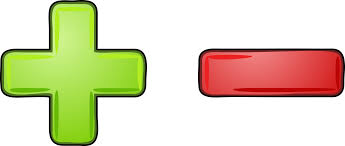1. Two and five are the only prime numbers in the entire number system which ends with two or five.

2. A geometrical close bounded figure which has 20 sides is known as an icosagon.

3. In the Indian number system, from 0 to 1000, the letter A only appears first in 1000 ("one thousand").

4. The calculator which is the most widely used mathematical device in today's world has actually originated from the concept of an abacus.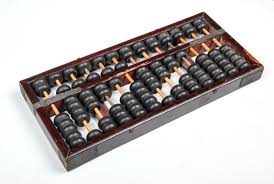1. If the sum of the digits is divisible by three, then the given number will also be divisible by three. This is known as the rule of divisibility of three.

2. If you add up all the numbers between 1 to 100 consecutively then the total that you will reach is 5050.

3. Jiffy is actually considered to be a unit of time for 1/100th of a second.1. #### Do you know what a Palindrome Number is? It is a number that reads the same backward and forward, e.g. 12421.

2. There are other special numbers also apart from Pythagoras constant and pi. Some of these numbers are Euler’s constant, Golden ratio, etc.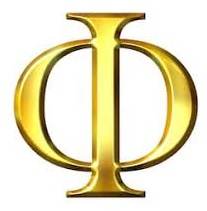1. The minimum number of cuts that are required to cut the figure into eight pieces are three i.e. horizontally, vertically, and cutting by the base.

2. The opposite sides of a dice always add up to 7. For example, 6 and 1 will always be on opposite sides which add up to 7.

3. A baseball field is of the perfect shape of a rhombus.## Conclusion

These math fun facts will help students get involved with this subject in a better way. These facts can also be used for various GK quizzes and questions. Apart from this, it can also be an interesting way to amaze your friends and become a Maths Guru.

Cuemath comes up with regular articles for parents and kids to address different topics. Our suggestions and recommendations are provided after extensive research and have helped a number of readers.

Can you come up with a few more facts?

Cuemath, a student-friendly mathematics and coding platform, conducts regular Online Live Classes for academics and skill-development, and their Mental Math App, on both iOS and Android, is a one-stop solution for kids to develop multiple skills. Understand the Cuemath Fee structure and sign up for a free trial.